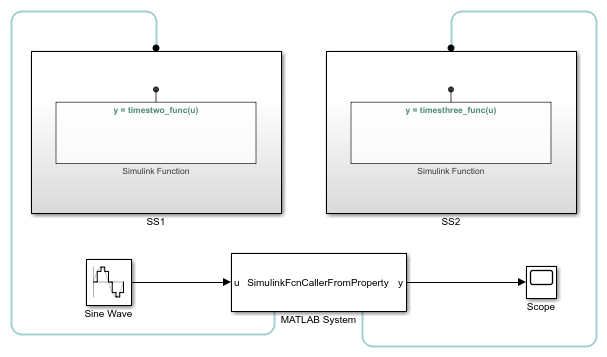## Call Simulink Functions from MATLAB System Block

A Simulink Function is a graphical representation of a computational unit in the Simulink® environment. Once you create the Simulink function, it can be executed by any computational unit and can be called in multiple places. You can only call a Simulink function inside the `stepImpl`, `outputImpl`, or `updateImpl` method of a System object™. See Simulink Functions Overview for more information on Simulink functions.

Note

`Interpreted mode` is not supported for a model that contains a MATLAB System block calling a Simulink Function.

### Create a Simulink Function Block

Set up a Simulink Function block that implements a simple function such as `y = 2 * u`.

2. When Function prototype window opens, type `y = timestwo_func(u)` as the function definition. This indicates that you define a function called `timestwo_func` that takes `u` as the input argument and produces `y` as the output argument. Alternatively, define the function name from Simulink Function block parameters.

3. Double-click the Simulink Function block and observe that a Trigger Port function block appears as well as an input and an output argument block.

4. Double-click the Trigger Port block in the Simulink Function, and observe that the Function visibility is set to `scoped`. To learn more about `scoped` Simulink Functions, see Scoped and Global Simulink Function Blocks Overview.

5. Add a Gain block and set its value to 2. Connect it with the input and output argument block. Click to return to the main model.

Note

To determine which Simulink Function a MATLAB System block is calling, turn on the function tracing lines. In the Debug tab, select Information Overlays > Function Connectors.

### Create a MATLAB System Block and Define System Object

Drag a MATLAB System block into the model and implement a System object to this block.

2. In the block dialog box, from the `New` list, select Basic. This opens a basic System object template for you to type your code.

3. To subclass an object from `matlab.System`, replace `Untitled` with the name of your System object. For this example, name it `SimulinkFcnCaller`.

4. In the `stepImpl` method of your System object, declare the Simulink Function using `getSimulinkFunctionNamesImpl`.

See an example of the System object code below. To learn more about how to write a System object, see Define Basic System Objects.

```classdef SimulinkFcnCaller < matlab.System % Public, tunable properties % SimulinkFcnCaller calls a Simulink Function from a % MATLAB System block to multiply the signal's value by 2. methods(Access = protected) function y = stepImpl(obj,u) % Implement algorithm. Calculate y as a function of input u and % discrete states. y = timestwo_func(u); end function names = getSimulinkFunctionNamesImpl(obj) % Use 'getSimulinkFunctionNamesImpl' method to declare % the name of the Simulink Function that will be called % from the MATLAB System block's System object code. names = {'timestwo_func'}; end end end```
5. Save the file and name it `SimulinkFcnCaller.m`.

### Call a Simulink Function in a Subsystem from a MATLAB System Block

The hierarchy of the Simulink Function block affects the function calls in the System object. For example, if the Simulink function is defined at a higher hierarchy in the Simulink model, the function is defined for all blocks in that hierarchy. If the Simulink function is defined at a lower hierarchy, you need to qualify the Subsystem and the function name. For example, suppose you have a Subsystem that contains a Simulink Function block at the same level as a MATLAB System block. When a Simulink Function block is placed in a subsystem, the function name is not visible to the outside the subsystem. You can call the Simulink Function block by qualifying the function name using the Subsystem name in your System object. To qualify the Subsystem and the function name follow these steps:

1. In the `stepImpl` method of your System object code, call the Simulink Function using dot notation. For example, in the code ```y = Subsystem1.timestwo_func(u)```, `Subsystem1` corresponds to the Subsystem, and `timestwo_func` corresponds to the Simulink Function name.

2. Similarly, declare the Subsystem and the Simulink Function in the `getSimulinkFunctionNamesImpl` method using the dot notation. The System object code shows the `timestwo` example written for a Simulink Function defined at a lower hierarchy than the MATLAB System block.

```classdef SimulinkFcnCallerQualified < matlab.System % SimulinkFcnCallerQualified calls a Simulink Function embedded in a Subsystem % from a MATLAB System block, and multiplies the signal's value by 2. methods(Access = protected) function y = stepImpl(obj,u) % Use the '.' notation to call a scoped Simulink Function from % a Simulink Function block. % Subsystem1 corresponds to the block name, where % timestwo_funct is the Simulink Function name. y = Subsystem1.timestwo_func(u); end function names = getSimulinkFunctionNamesImpl(obj) % Use the 'getSimulinkFunctionNamesImpl' method with the '.' % notation to declare the name of a Simulink Function in % MATLAB System block's System object code. names = {'Subsystem1.timestwo_func'}; end end end```

3. Save the System object file and name it `SimulinkFcnCallerQualified.m`.

### Call Simulink Functions from a MATLAB System Block

This example shows two `Simulink Functions` conditionally called by a `MATLAB System` block using the nontunable properties of the System object®.

The `MATLAB System` block calls one of the `Simulink Functions` inside two different subsystems, depending on the value of the signal coming from the `Sine Wave` block. If the value of the signal is less than 10, the `MATLAB System` block calls the `timestwo_func` `Simulink Function` inside the `SS1` `Subsystem` block. If the value is larger than 10, it calls the `timesthree_func` in the `SS2 Subsystem` block.

Function names are defined as nontunable properties, are switched from string to functions using the `str2func` function. Then, these functions are declared as properties in the `getSimulinkFunctionNamesImpl` method.